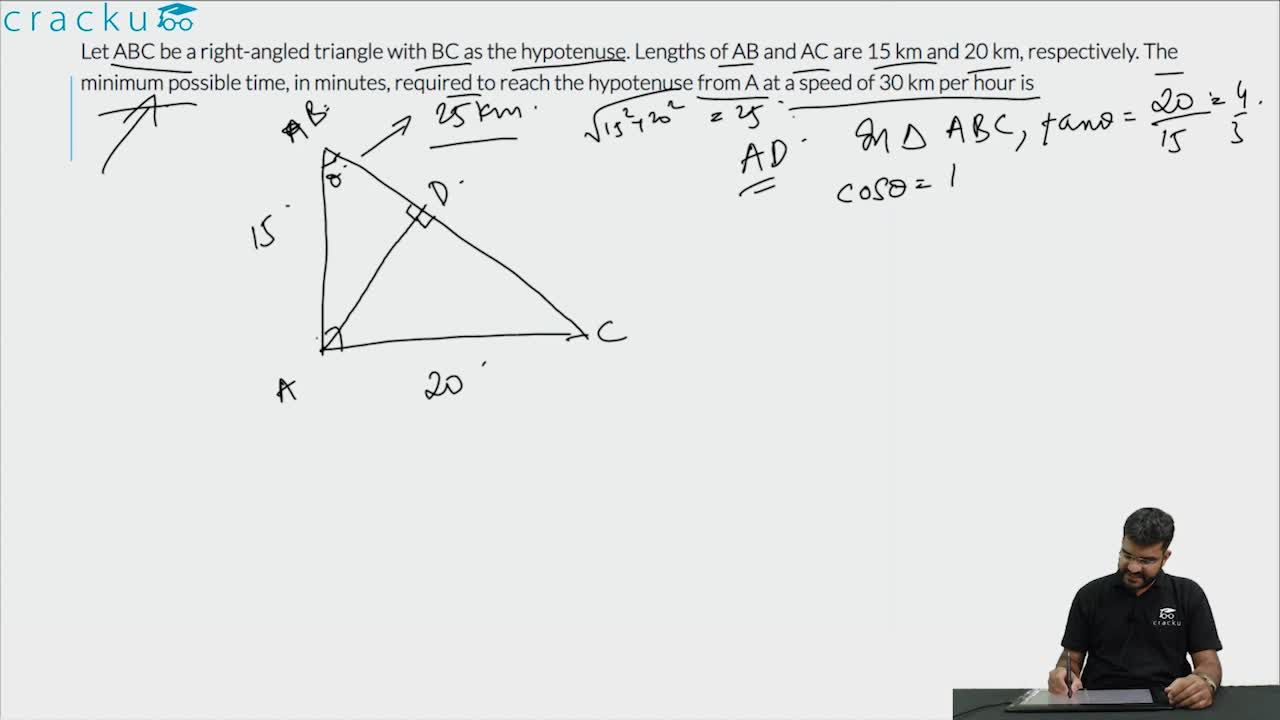Question 86

# Let ABC be a right-angled triangle with BC as the hypotenuse. Lengths of AB and AC are 15 km and 20 km, respectively. The minimum possible time, in minutes, required to reach the hypotenuse from A at a speed of 30 km per hour is

Solution

The length of the altitude from A to the hypotenuse will be the shortest distance.

This is a right triangle with sides 3 : 4 : 5.

Hence, the hypotenuse = $$\sqrt{20^2+15^2}$$ = 25 Km.

Length of the altitude = $$\frac{15 * 20}{25}$$ = 12 Km

(This is derived from equating area of triangle, $$\frac{15\cdot20}{2}\ =\ \frac{25\cdot\text{altitude}}{2}$$)

The time taken = $$\frac{12}{30} * 60$$ = 24 minutes

### View Video Solution• All Quant CAT Formulas and shortcuts PDF
• 30+ CAT previous papers with solutions PDF

##### Ayushi Choudhary

3 years ago

Why can't I use the area formula? I can calculate the area of ∆ABC using 1/2*ab*sin90 (as it's a right angled triangle) and then put the calculated area equals to 1/2*b*h. Thus, I will get AD (as it's the height of the ∆) by doing so, I get 15km as h and my answer doesn't match. Why is this formula wrong?